# transformer详解：transformer/ universal transformer/ transformer-XL

Attention机制在NLP领域的应用最早可以追朔到2014年，Bengio团队将Attention引入NMT(神经机器翻译)任务 。之后更是在深度学习的各个领域得到了广泛应用：如CV中用于捕捉图像上的感受野；NLP中定位关键token/feature.

• Transformer
首个完全抛弃RNN的recurrence，CNN的convolution，仅用attention来做特征抽取的模型
• Universal Transformer
重新将recurrence引入transformer，并加入自适应的思想，使得transformer图灵完备，并有着更好的泛化性和计算效率
• Transformer-XL
在transformer的基础上加入Segment-level Recurrence和相对位置编码，从而可以处理超长输入序列，并且更加高效。

1. 为什么要引入attention机制？
Attention机制理论上可以建模任意长度的长距离依赖，并且符合人类直觉
2. transformer有哪些优点和不足？
完全基于attention，在可以并行的情况下仍然有很强的特征抽取能力，缺点是仍然是自回归的形式、理论上非图灵完备、缺少Recurrent Inductive Bias和条件计算、对超长序列建模能力较差
3. universal transformer相较于transformer做了哪些改进，有什么不足？
利用Recurrence将transformer的层数由6改为了任意层，并且理论上实现了图灵完备（指的是计算上通用）；加入自适应计算的思想，使得模型计算更加高效。
4. transformer-XL的特点，优势是什么？
引入Segment-level Recurrence，使得transformer可以解决“上下文碎片问题”并捕捉更长距离的依赖，从而可以处理超输入序列

transformer仍然是基于seq2seq，所以介绍attention机制之前，需要先介绍下“前attention”时代，NLP领域中的seq2seq。

## seq2seq简介

### RNN-based seq2seq

seq2seq最早来源于通信，对应过程中的编码和解码。在NLP领域中，seq2seq主要用来解决将一个序列X转化为另一个序列Y这一类问题，通常应用于机器翻译、自动摘要等端到端的生成式应用。seq2seq有一个很重要的特点：输入序列X和输出序列Y可以不等长，不需要一一对应。

P(Y | X)=\sum_{t=1}^{T} \log P\left(y_{t} | y_{1 : t-1}, X\right)

• X为输入序列
• T代表时刻，y_{1 : t-1}则代表decoder前t-1时间的输出
1. 对于seq2seq而言，在训练时y_{1 : t-1}是ground truth tokens；而在测试的时候，没有ground truth tokens，此时是采用decoder生成的y’_{1 : t-1}来预测下一个词。训练和预测时这种不一致，也是seq2seq中长期存在的“暴露偏差”的根源，针对这一问题，也有很多的工作，这里就不再展开。
2. 上述过程预测输出序列Y的token时，是根据之前时刻的结果y’_{1 : t-1}来预测下一个token，即极大化下一目标token的概率，这是一种典型的贪心策略，得到的是局部最优；而对于一般的端到端生成模型而言，希望得到整个序列的最佳，即最后的生成序列Y的tokens顺序排列的联合概率最大，找到一个全局最优。实际过程中seq2seq在预测时并不使用这种greedy search的策略，而是采用beam search。不过本文主要讲述transformer，seq2seq仅做一个便于问题理解的概述。Encoder会将输入序列X编码为一个固定长度的语义向量C：在编码过程中，时刻t的输出仅依赖前一时刻隐层h_{t-1} 和当前时刻的输入x_{t}
Decoder的输入是encoder得到的语义向量C：解码过程中，时刻t的输出依赖于三个部分，上一个时刻隐层状态h_{t-1}和中间语义向量C和上一个时刻的预测输出y_{t-1}
Seq2Seq两个部分(Encoder和Decoder)联合训练的目标函数是最大化条件似然函数：
\max _{\boldsymbol{\theta}} \frac{1}{N} \sum_{n=1}^{N} \log p_{\boldsymbol{\theta}}\left(\mathbf{y}_{n} | \mathbf{x}_{n}\right)

seq2seq中encoder的输入x_i和decoder中的输出y_i都是词的高维向量表示，向量中蕴涵一定的语义信息，之所以可以用一个高维向量来表示词的语义，其基本假设是上世纪语言学家提出的分布式语义的思想：其认为一个词可以由上下文中的词来表示。

• 如果我们试图预测“the clouds are in the sky”中的最后一个单词，我们不需要任何更多的上下文信息，从”clonds”, “in” 这些近距离的词就可以预测到下一个单词是“sky”
• 而如果尝试预测“I grew up in France… … I speak fluent French.”中的”French”，那么需要重点关注远距离的”France”。

### RNN-based seq2seq with attention

RNN在将一个不定长输入序列转化为定长的中间语义向量时，会有信息损失，为了避免这样的损失，就引入了Attention机制。Attention机制本身有些类似于人类的直观活动：人在观察一副图画时，直觉会重点关注“更为重要”的信息；人类译者在做翻译时，也会更侧重当前翻译部分所对应的上下文。基于这样的原理，Attention机制在做机器翻译时，会尝试关注源语句中“更为重要”信息，再结合已经翻译的部分，最终得出当前译文。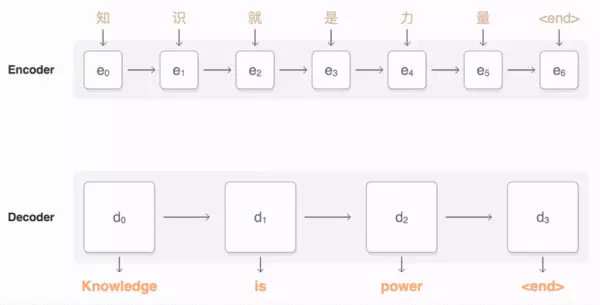## Transformer

• Encoder：读取输入语句并生成其representation。
• Decoder：参考Encoder生成的输入语句的representation，逐词生成输出语句。

transformer首先为每个单词生成初始表示或使用预先定义的wordembedding，动图中用空白的圆表示；然后通过self attention，从所有其他单词中收集信息，生成一个新的representation，每个单词由整个上下文(动图中由填满的圆表示)的信息来表示。然后，对所有单词并行重复此步骤多次，依次生成新的representation。Encoder：由6个相同的层堆叠而成，每层有两个sub layer：multi-head self-attention层和一个全连接前馈神经网络；sub layer之间经layer normalization后，再通过residual connection连接。

### Attention

#### Scaled Dot-Product Attention

Self Attention本质上是将上下文词的representation加权求和作为当前词的representation。需要关注的部分是我们如何得到加权求和的权值。在Transformer中，这一部分用Attention(Q,K,V)来体现 ，Q是query，K是Key；通过Q和K的点积的结果来体现上下文词对当前词的影响程度，再通过softmax得到归一化权重：
\text { Attention }(Q, K, V)=\operatorname{softmax}\left(\frac{Q K^{T}}{\sqrt{d_{k}}}\right) V

\sqrt{d_{k}}是一个缩放因子，d_k是向量Q_i,V_i,K_i的维度，因为Q_iK_i^T \in (0,d_k)，当d_k比较大时，Q_iK_i^T值可能会很大，softmax之后可能会出现较多的值接近0。下图是Scaled Dot-Product Attention的示意图：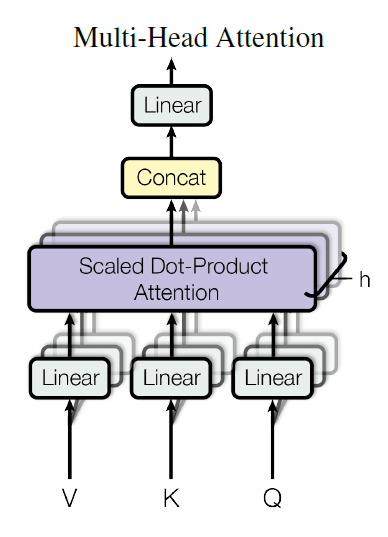\begin{aligned} \text { MultiHead }(Q, K, V) &=\text { Concat }\left(\text { head }_{1}, \ldots, \text { head }_{\mathrm{h}}\right) W^{O}\\\text { where head }_{\mathrm{i}} &=\text { Attention }\left(Q W_{i}^{Q}, K W_{i}^{K}, V W_{i}^{V}\right) \end{aligned}

• 可以综合各个单词，在不同表示子空间的信息

#### attention in transformer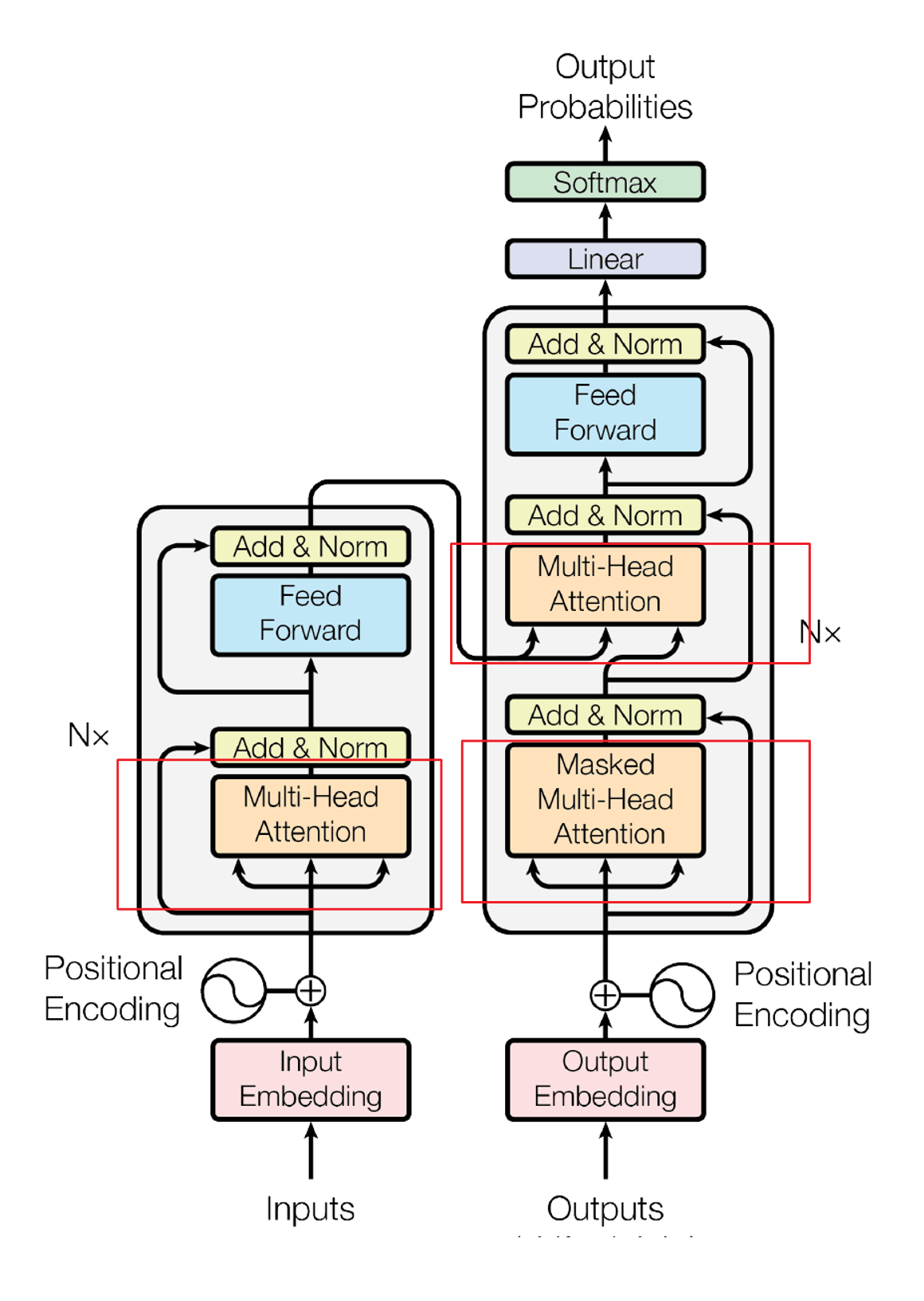### Position-wise Feed-Forward Networks

decoder和encoder中都存在一个相同的全连接前馈神经网络，该网络包括两次线性变换，两次变换中间是一个ReLU激活函数
\operatorname{FFN}(x)=\max \left(0, x W_{1}+b_{1}\right) W_{2}+b_{2}

• 各个层中的网络结构相同，但是参数不同
• 输入和输出的向量维度均为512，中间层的维度是2048

### Positional Encoding

\begin{aligned} P E_{(p o s, 2 i)} &=\sin \left(\operatorname{pos} / 10000^{2 i / d_{\text {model }}}\right)\\P E_{(\text {pos, } 2 i+1)} &=\cos \left(\text { pos } / 10000^{2 i / d_{\text {model }}}\right) \end{aligned}

\begin{array}{c}{P E(p o s+k, 2 i)=P E(p o s, 2 i) P E(k, 2 i+1)+P E(p o s, 2 i+1) P E(k, 2 i)}\\{P E(p o s+k, 2 i+1)=P E(p o s, 2 i+1) P E(k, 2 i+1)-P E(p o s, 2 i) P E(k, 2 i)}\end{array}

### bag of tricks

transformer中还有一些细节，碎碎念：Transformer的细枝末节这篇文章已经写得很棒，就不再赘述。

• Weight Tying
• Learning rate warm-up
• Regularization

### 缺陷

transformer一经提出，在NLP领域也引起了广泛关注，在当下（2019年）仍有很多任务的STOA方法是基于transformer的。当然transformer也存在着不足：

• 缺少Recurrent Inductive Bias
学习算法中Inductive Bias可以用来预测从未遇到的输入的输出（参考)。对于很多序列建模任务（如需要对输入的层次结构进行建模时，或者在训练和推理期间输入长度的分布不同时），Recurrent Inductive Bias至关重要。EMNLP 2018 上的一个工作对这一点进行了实证。
• Transformer是非图灵完备的
非图灵完备通俗的理解，就是无法解决所有的问题（可以参考)
在Transformer中，单层中sequential operation (context two symbols需要的操作数)是O(1) time，独立于输入序列的长度。那么总的sequenctial operation仅由层数T决定。这意味着transformer不能在计算上通用，即无法处理某些输入。如：输入是一个需要对每个输入元素进行顺序处理的函数，在这种情况下，对于任意给定的深度T的transformer，都可以构造一个长度为N > T的输入序列，该序列不能被transformer正确处理
• transformer缺少conditional computation
transformer在encoder的过程中，所有输入元素都有相同的计算量，比如对于“I arrived at the bank after crossing the river”, 和”river”相比，需要更多的背景知识来推断单词”bank”的含义，然而transformer在编码这个句子的时候，无条件对于每个单词应用相同的计算量，这样的过程显然是低效的。
• 不能很好的处理超长输入
理论上来说，attention可以关联两个任意远距离的词，但实际中，由于计算资源有限，仍然会限制输入序列的长度，超过这个长度的序列会被截断。

## Universal Transformeruniversal transformer第二个比较大的改进是加入了dynamic halting: 基于自适应时间算法(Adaptive Computation Time, ACT) ，该算法由DeepMind在2016年提出。有了dynamic halting，在编码“I arrived at the bank after crossing the river”, 对于”river”编码的递归次数会变少；对于“bank”的编码递归次数会相应更多.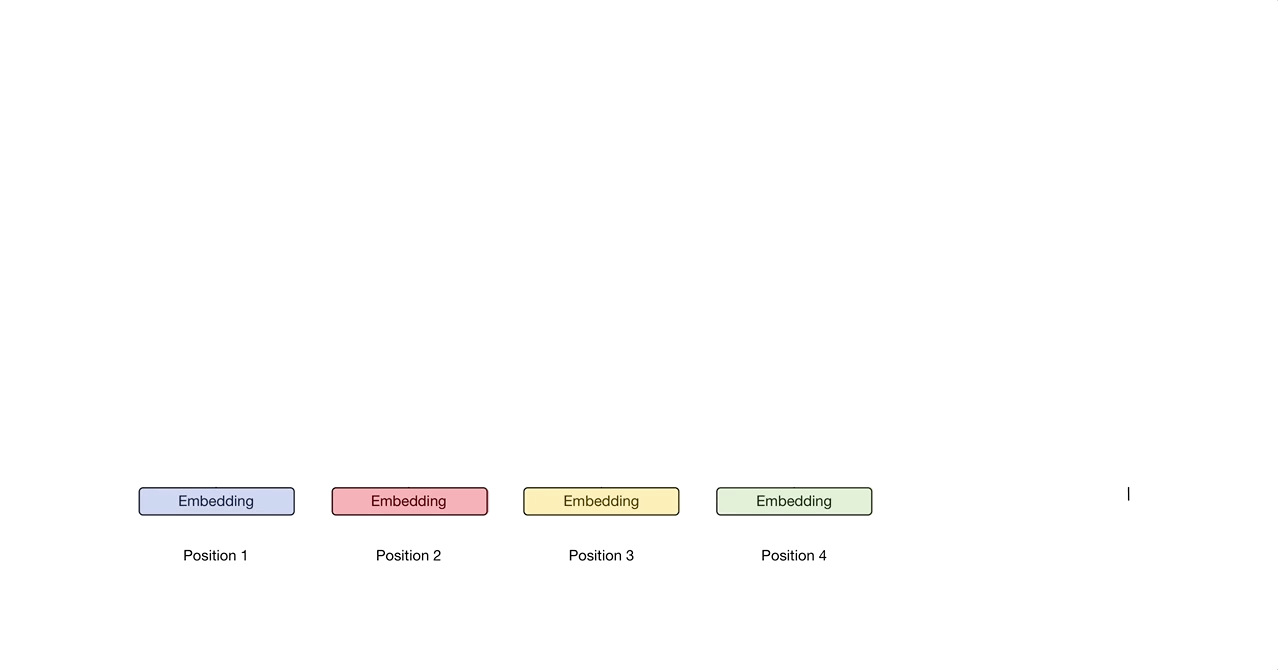## transformer-XL

• 能建模的依赖关系不会超过segment的长度
• 会导致context fragmentation（上下文碎片化）：因为分片并不是根据语义边界，而是根据长度划分，很有可能会将一个完整的句子分割，那么在预测一个segment的前几个token的时候，很可能缺乏必要的语义信息。

### Segment-level Recurrence### Relative Positional Encodeing

\begin{aligned} \mathbf{h}_{\tau+1} &=f\left(\mathbf{h}_{\tau}, \mathbf{E}_{\mathbf{s}_{\tau+1}}+\mathbf{U}_{1: L}\right)\\\mathbf{h}_{\tau} &=f\left(\mathbf{h}_{\tau-1}, \mathbf{E}_{\mathbf{s}_{\tau}}+\mathbf{U}_{1: L}\right) \end{aligned}

\begin{aligned} \mathbf{A}_{i, j}^{\mathrm{abs}} &=\underbrace{\mathbf{E}_{x_{i}}^{\top} \mathbf{W}_{q}^{\top} \mathbf{W}_{k} \mathbf{E}_{x_{j}}}_{(a)}+\underbrace{\mathbf{E}_{x_{i}}^{\top} \mathbf{W}_{q}^{\top} \mathbf{W}_{k} \mathbf{U}_{j}}_{(b)}\\&+\underbrace{\mathbf{U}_{i}^{\top} \mathbf{W}_{q}^{\top} \mathbf{W}_{k} \mathbf{E}_{x_{j}}}_{(c)}+\underbrace{\mathbf{U}_{i}^{\top} \mathbf{W}_{q}^{\top} \mathbf{W}_{k} \mathbf{U}_{j}}_{(d)} \end{aligned}

W_q{E}_{x_{i}}得到的是第i个token的Query向量，W_k{E}_{x_{j}}得到的是第j个token的key向量，U_i代表第i个token的位置编码，所以：

• a是query向量和key向量的相乘
• b是query向量和key对应的位置编码相乘
• c是query对应位置编码和key向量相乘
• d是query对应位置编码和key对应位置编码相乘

\begin{aligned} \mathbf{A}_{i, j}^{\mathrm{rel}} &=\underbrace{\mathbf{E}_{x_{i}}^{\top} \mathbf{W}_{q}^{\top} \mathbf{W}_{k, E} \mathbf{E}_{x_{j}}}_{(a)}+\underbrace{\mathbf{E}_{x_{i}}^{\top} \mathbf{W}_{q}^{\top} \mathbf{W}_{k, R} \mathbf{R}_{i-j}}_{(b)}\\&+\underbrace{u^{\top} \mathbf{W}_{k, E} \mathbf{E}_{x_{j}}}_{(c)}+\underbrace{v^{\top} \mathbf{W}_{k, R} \mathbf{R}_{i-j}}_{(d)} \end{aligned}

• b和d中将Key的绝对位置编码U_j改为了相对位置编码R_{i-j}
R_{i-j}是一个无需学习的sinusoidal编码矩阵，计算方式同最初的transformer。该方式可以避免不同segments之间由于tokens在各自segment的index相同而产生的时序冲突的问题。
• c和d中，将query的绝对位置编码进行了替换
因为query相对于自己的位置是一样，a那么ttention bias的计算与query在序列中的绝对位置无关，应当保持不变
• $W_k$->$W_{k,E},W_{k,R}$
在之前transformer中，token的embedding和position embedding会加起来，再经过$W_k$矩阵做线性变换，即embedding和position encoing是相同的线性变换，该处改变使得key的embedding和positional encoding 分别采用了不同的线性变换。其中W_{k,E}对应于key的embedding线性映射矩阵，W_{k,R}对应与key的positional encoding的线性映射矩阵

• 解决了在应用了Segment-level Recurrence之后，segment间位置编码冲突的问题
• 可以利用sinusoid 的inductive bias
sinusoid并不会受限于序列长度，即使是训练时从未遇到的序列长度仍能很好的处理，这也一定程度上体现了inductive bias

### Faster Evaluation

Vanilla transformer在评估时，每个segment只会向前移动一个token的位置，这样的速度很慢：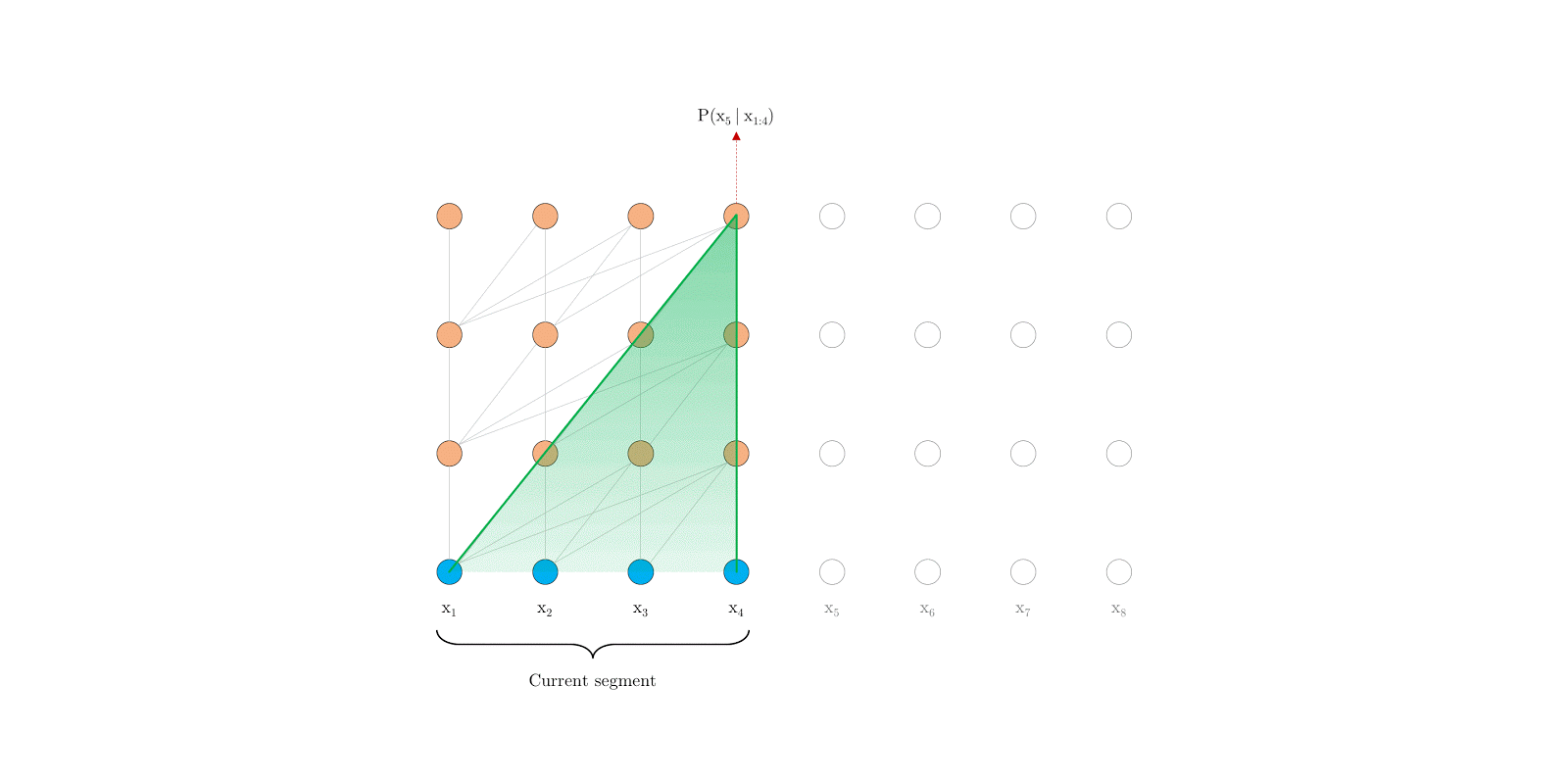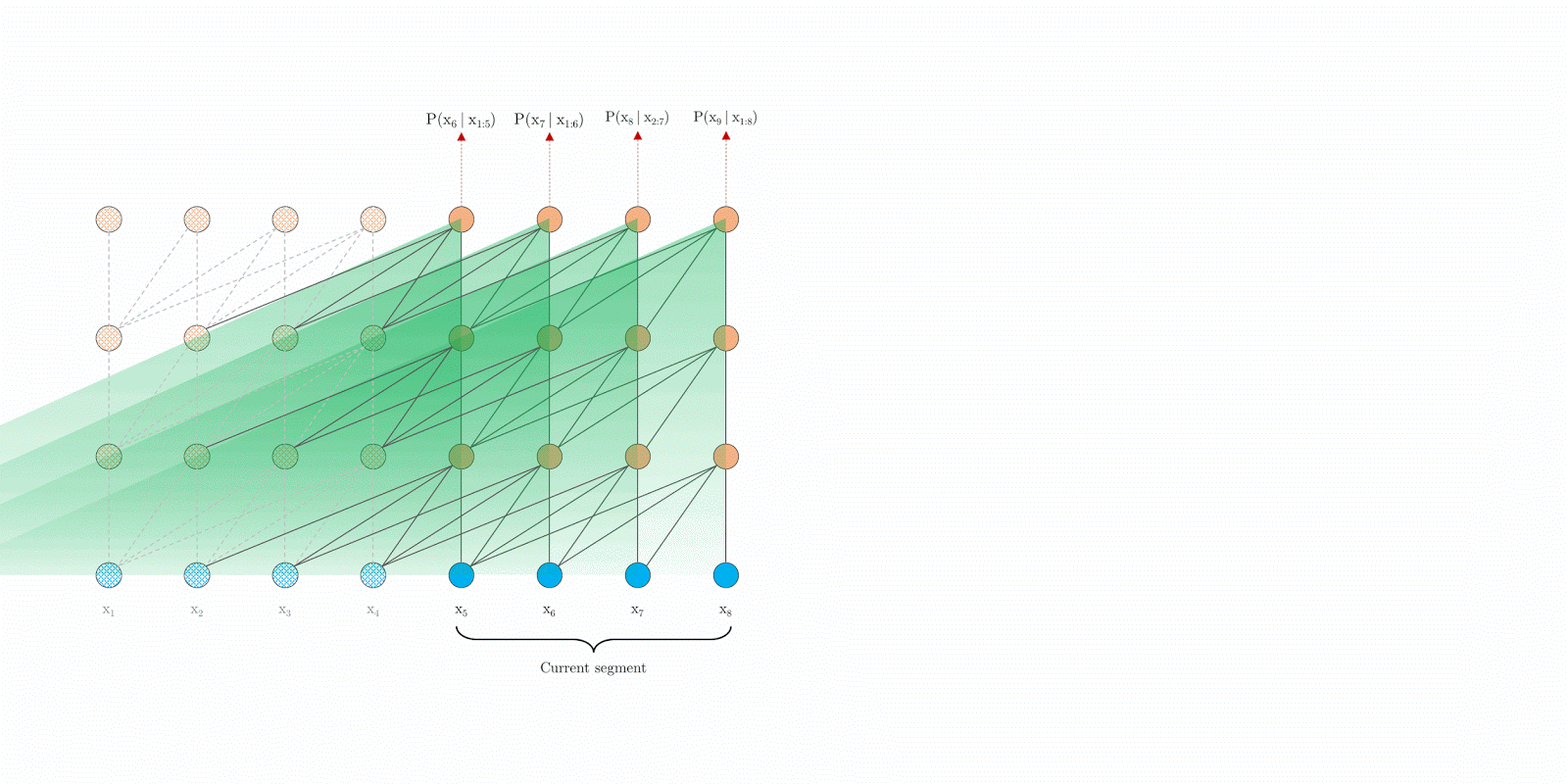### Results

• Transformer-XL学习的依赖项比RNNs长80%左右，比最初的transformer长450%，最初的transformer通常比RNNs具有更好的性能，但由于上下文的长度固定，不是远程依赖项建模的最佳选择
• 在评估语言建模任务时，ransformer-XL的速度比vanilla transformer快1800多倍，因为不需要重新计算（见Faster Evaluation部分）
• 由于有更好长距离依赖建模，Transformer-XL在长序列上具有更好的perplexity性能(更准确地预测样本)；而且通过解决上下文碎片问题，它在短序列上也有更好的性能。